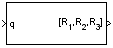Quaternions to Rotation Angles

Determine rotation vector from quaternion

• Library:
• Aerospace Blockset / Utilities / Axes Transformations

•Description

The Quaternions to Rotation Angles block converts the four-element quaternion vector (q0, q1, q2, q3), into the rotation described by the three rotation angles (R1, R2, R3). The block generates the conversion by comparing elements in the direction cosine matrix (DCM) as a function of the rotation angles. The rotation used in this block is a passive transformation between two coordinate systems. The elements in the DCM are functions of a unit quaternion vector. Aerospace Blockset™ uses quaternions that are defined using the scalar-first convention. This block normalizes all quaternion inputs. For more information on the direction cosine matrix, see Algorithms.

Limitations

• For the ZYX, ZXY, YXZ, YZX, XYZ, and XZY rotations, the block generates an R2 angle that lies between ±pi/2 radians, and R1 and R3 angles that lie between ±pi radians.

• For the 'ZYZ', 'ZXZ', 'YXY', 'YZY', 'XYX', and 'XZX' rotations, the block generates an R2 angle that lies between 0 and pi radians, and R1 and R3 angles that lie between ±pi radians. However, in the latter case, when R2 is 0, R3 is set to 0 radians.

Ports

Input

expand all

Quaternion, specified as a 4-by-1 vector.

Data Types: double

Output

expand all

Rotation angles, returned 3-by-1 vector, in radians.

Data Types: double

Parameters

expand all

Output rotation order for the three rotation angles.

Programmatic Use

 Block Parameter: rotationOrder Type: character vector Values: ZYX | ZYZ |ZXY | ZXZ | YXZ | YXY | YZX | YZY | XYZ | XYX | XZY | XZX Default: 'ZYX'

Algorithms

The elements in the DCM are functions of a unit quaternion vector. For example, for the rotation order z-y-x, the DCM is defined as:

$DCM=\left[\begin{array}{lll}\mathrm{cos}\theta \mathrm{cos}\psi \hfill & \mathrm{cos}\theta \mathrm{sin}\psi \hfill & -\mathrm{sin}\theta \hfill \\ \left(\mathrm{sin}\varphi \mathrm{sin}\theta \mathrm{cos}\psi -\mathrm{cos}\varphi \mathrm{sin}\psi \right)\hfill & \left(\mathrm{sin}\varphi \mathrm{sin}\theta \mathrm{sin}\psi +\mathrm{cos}\varphi \mathrm{cos}\psi \right)\hfill & \mathrm{sin}\varphi \mathrm{cos}\theta \hfill \\ \left(\mathrm{cos}\varphi \mathrm{sin}\theta \mathrm{cos}\psi +\mathrm{sin}\varphi \mathrm{sin}\psi \right)\hfill & \left(\mathrm{cos}\varphi \mathrm{sin}\theta \mathrm{sin}\psi -\mathrm{sin}\varphi \mathrm{cos}\psi \right)\hfill & \mathrm{cos}\varphi \mathrm{cos}\theta \hfill \end{array}\right]$

The DCM defined by a unit quaternion vector is:

$DCM=\left[\begin{array}{lll}\left({q}_{0}^{2}+{q}_{1}^{2}-{q}_{2}^{2}-{q}_{3}^{2}\right)\hfill & 2\left({q}_{1}{q}_{2}+{q}_{0}{q}_{3}\right)\hfill & 2\left({q}_{1}{q}_{3}-{q}_{0}{q}_{2}\right)\hfill \\ 2\left({q}_{1}{q}_{2}-{q}_{0}{q}_{3}\right)\hfill & \left({q}_{0}^{2}-{q}_{1}^{2}+{q}_{2}^{2}-{q}_{3}^{2}\right)\hfill & 2\left({q}_{2}{q}_{3}+{q}_{0}{q}_{1}\right)\hfill \\ 2\left({q}_{1}{q}_{3}+{q}_{0}{q}_{2}\right)\hfill & 2\left({q}_{2}{q}_{3}-{q}_{0}{q}_{1}\right)\hfill & \left({q}_{0}^{2}-{q}_{1}^{2}-{q}_{2}^{2}+{q}_{3}^{2}\right)\hfill \end{array}\right]$

From the preceding equation, you can derive the following relationships between DCM elements and individual rotation angles for a ZYX rotation order:

$\begin{array}{c}\varphi =\text{atan}\left(DCM\left(2,3\right),DCM\left(3,3\right)\right)\\ =\text{atan}\left(2\left({q}_{2}{q}_{3}+{q}_{0}{q}_{1}\right),\left({q}_{0}^{2}-{q}_{1}^{2}-{q}_{2}^{2}+{q}_{3}^{2}\right)\right)\\ \theta =\text{asin}\left(-DCM\left(1,3\right)\right)\\ =\text{asin}\left(-2\left({q}_{1}{q}_{3}-{q}_{0}{q}_{2}\right)\right)\\ \psi =\text{atan}\left(DCM\left(1,2\right),DCM\left(1,1\right)\right)\\ =\text{atan}\left(2\left({q}_{1}{q}_{2}+{q}_{0}{q}_{3}\right),\left({q}_{0}^{2}+{q}_{1}^{2}-{q}_{2}^{2}-{q}_{3}^{2}\right)\right)\end{array}$

where Ψ is R1, Θ is R2, and Φ is R3.

Extended Capabilities

C/C++ Code GenerationGenerate C and C++ code using Simulink® Coder™.

Introduced in R2007b

Aerospace Blockset DocumentationGet trial now